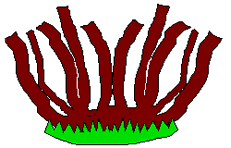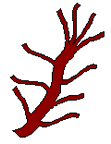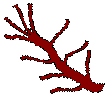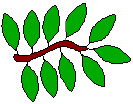#### You may also like### Chocolate

There are three tables in a room with blocks of chocolate on each. Where would be the best place for each child in the class to sit if they came in one at a time?### Four Triangles Puzzle

Cut four triangles from a square as shown in the picture. How many different shapes can you make by fitting the four triangles back together?### Cut it Out

Can you dissect an equilateral triangle into 6 smaller ones? What number of smaller equilateral triangles is it NOT possible to dissect a larger equilateral triangle into?

# The Deca Tree

## The Deca Tree

In the forest there is a Deca Tree.
A Deca Tree has $10$ trunks,and on each trunk there are $10$ branches,and on each branch there are $10$ twigs,and on each twig there are $10$ leaves:One day a woodcutter came along and cut down one trunk from the tree.
Then he cut off one branch from another trunk of the tree.
Then he cut off one twig from another branch.
Finally he pulled one leaf from another twig.

How many leaves were left on the tree then?

### Why do this problem?

This problem can give some substance to children's ideas on place value especially when you are introducing or using numbers greater than $1000$. It shows in a pictorial way what the extra zeros do to a number when multiplying by $10$ and $100$.

The problem can also help children to appreciate how big large numbers really are!

### Possible approach

You could introduce the problem by telling the class the 'story' about the tree and its trunks, its branches, its twig and its leaves. During, or after this, ask such questions as "How many branches are there altogether?" and "How many leaves will there be on each branch?".

Then, working in pairs, the children could work out the details of the problem. It might help them to draw rough sketches and/or make a table/list of the numbers of the trunks, branches, twigs and leaves, both at the beginning and after the woodcutter has been at work.

After the number of leaves taken off the tree has been calculated this needs to be subtracted from the total of all the leaves on the tree. This might be the time for a plenary so that you work on the calculation together as as class.

Some children may have do the problem differently, such as deducting the leaves removed from the tree as they go. This could lead to an interesting discussion on different methods to tackling problems such as this.

### Key questions

If there are ten twigs on each branch, how many will there be on ten branches?
How many leaves are there on ten twigs?
How many leaves did the Deca Tree have before the woodcutter came along?
How many leaves did the woodcutter chop off each time?

### Possible extension

Children could be invited to invent a similar tree with different numbers of branches etc. such a five-branched 'Penta' tree or an eight-branched 'Octa' tree.

### Possible support

In addition to, or instead of, drawing sketches, it might help some children to make a model of the tree, or at least part of the tree. Pipecleaners could work well for this purpose.